StatLect

# Unbiased estimator

An estimator of a given parameter is said to be unbiased if its expected value is equal to the true value of the parameter.

In other words, an estimator is unbiased if it produces parameter estimates that are on average correct.## Definition

Remember that in a parameter estimation problem:

• we observe some data (a sample, denoted by), which has been extracted from an unknown probability distribution;

• we want to estimate a parameter(e.g., the mean or the variance) of the distribution that generated our sample;

• we produce an estimate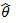of(i.e., our best guess of) by using the information provided by the sample.

The estimate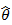is usually obtained by using a predefined rule (a function) that associates an estimateto each samplethat could possibly be observed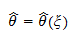The function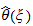is called an estimator.

Definition An estimatoris said to be unbiased if and only if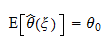where the expected value is calculated with respect to the probability distribution of the sample.

## Examples

The following table contains examples of unbiased estimators (with links to lectures where unbiasedness is proved).

Estimator Estimated parameter Lecture where proof can be found
Sample mean Expected value Estimation of the mean
Adjusted sample variance Variance Estimation of the variance
OLS estimator Linear regression coefficients Gauss-Markov theorem
Adjusted sample variance of the OLS residuals Variance of the error of a linear regression Normal linear regression model

## Biased estimator

An estimator which is not unbiased is said to be biased.

## Bias

The bias of an estimatoris the expected difference betweenand the true parameter: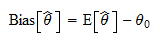Thus, an estimator is unbiased if its bias is equal to zero, and biased otherwise.

## More details

Unbiasedness is discussed in more detail in the lecture entitled Point estimation.

## Keep reading the glossary

Previous entry: Unadjusted sample variance

Next entry: Variance formula

## How to cite

Taboga, Marco (2021). "Unbiased estimator", Lectures on probability theory and mathematical statistics. Kindle Direct Publishing. Online appendix. https://www.statlect.com/glossary/unbiased-estimator.

The books

Most of the learning materials found on this website are now available in a traditional textbook format.

Featured pages
Explore
Glossary entries
Share
• To enhance your privacy,
• we removed the social buttons,
• but don't forget to share.